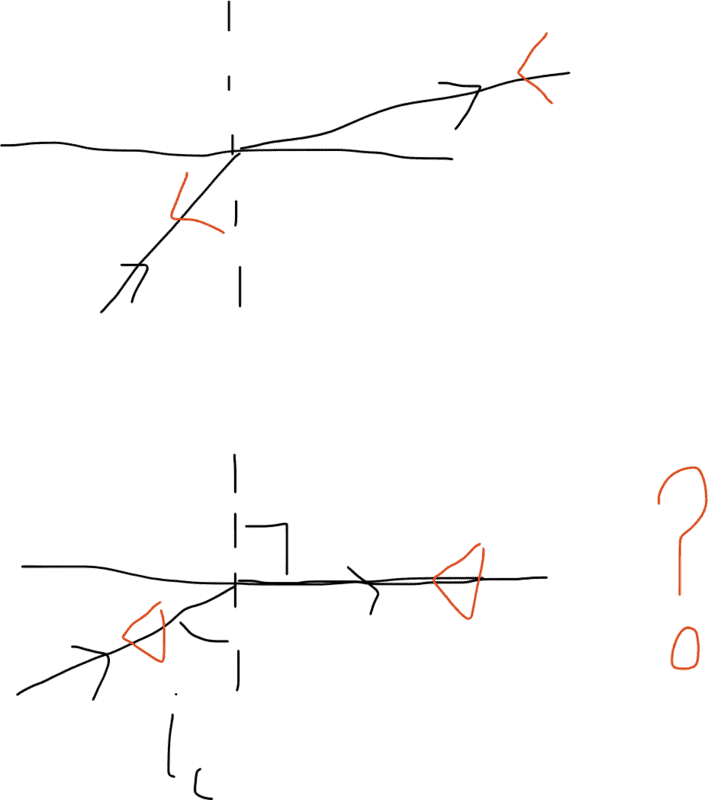# Critical angle (symmetry of refraction) confusion

Glenn GRefraction has a symmetry; on going from glass to air light at the glass air surfaces refracts away from the normal. If you turn the light source around and make the former refracted beam the incident beam then its refracted angle will be the former incident angle. So at critical angle, do the same trick, skim the light along the surface. how does it know what to do? carry on skimming or refract into the glass at 41.8 degrees.

what then troubled me even more is that if a beam skimming the surface can spontaneously refract into the glass the why doesn't light refracted at 90 degrees (due to i being critical) refracted back in again .

Does this mean that the whole idea of a critical angle is a limiting idea ... if below critical light refracts out the block, if incident angle is above the critical we get TIR - so what actually happens at the boundary between these two?

Homework Helper
Gold Member
I think you have it right, that it is a limit type of behavior as the critical angle is approached. The reflectivity is high, near unity, as the light skims the surface on the air side. Measuring a precise angle of refraction becomes more difficult, as the incident beam skims the surface.

•Glenn G
Homework Helper
2022 Award
Notice that there is no real discontinuity here however. Why would you expect the glancing beam to suddenly snap to 90 deg?
Only a 0deg entrant beam exits at 0 deg. The angles and intensities are smooth functions. I guess "total internal reflection" is a bit surprising.
Edited

Last edited:
•Glenn G
Glenn G
Yeah, still baffled by the skimming on the surface reality. Feels like we've either got refraction away or reflection off but if you drill at the interface between refraction away and reflection don't think you can wave the 90% refracted angle away. Refracted angle is clearly approaching 90 degrees as i is increasing and at some point we do get TIR ... both frustrated and fascinated by this in equal measure

Glenn G
I think you have it right, that it is a limit type of behavior as the critical angle is approached. The reflectivity is high, near unity, as the light skims the surface on the air side. Measuring a precise angle of refraction becomes more difficult, as the incident beam skims the surface.
Sorry I meant the glancing beam starts to refract in at 41.8 degrees - if we reverse the arrows in the above diagram